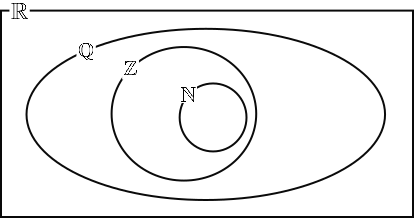# Exam-Style Questions on Number Systems

## Problems on Number Systems adapted from questions set in previous Mathematics exams.

### 1.

IB Studies

This Venn diagram shows the relationship between the sets of numbers

$$\mathbb N, \mathbb Z, \mathbb Q \text{ and } \mathbb R$$Write down the following numbers in the appropriate place in the Venn diagram.

(a) $$5$$

(b) $$\frac23$$

(c) $$\pi$$

(d) $$0.91$$

(e) $$\sqrt 7$$

(f) $$-0.75$$

If you would like space on the right of the question to write out the solution try this Thinning Feature. It will collapse the text into the left half of your screen but large diagrams will remain unchanged.

The exam-style questions appearing on this site are based on those set in previous examinations (or sample assessment papers for future examinations) by the major examination boards. The wording, diagrams and figures used in these questions have been changed from the originals so that students can have fresh, relevant problem solving practice even if they have previously worked through the related exam paper.

The solutions to the questions on this website are only available to those who have a Transum Subscription.

Exam-Style Questions Main Page

Search for exam-style questions containing a particular word or phrase:

To search the entire Transum website use the search box in the grey area below.